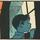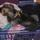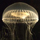## Social Question# Is it obvious that (a+c)/(b+d) is between a/b and c/d?

Asked by LostInParadise (27413) July 8th, 2019

For positive whole numbers a, b, c and d. The result is called the mediant theorem, and I have seen it described as non-intuitive.

What if it is framed as follows? A team plays b games at the start of the season and wins a of them. Out of the remaining d games, it wins c. The winning rate for the whole season, (a+c)/(b+d), must be between the winning rate for the start of the season, a/b, and the wining rate for the end of the season, c/d.

Here is another sports application. Going into a game, a baseball player is batting .300. In the game, the player goes one for four, which must lower his average.

Observing members: 0Composing members: 0No it isn’t obvious, because one does not know the magnitude of the ratios, and also one does not know the relationship between the numerators and denominators.

zenvelo (35041)“Great Answer” (2) Flag as…let’s put some numbers in place
a=2
b=1
c=6
d=3
so (a+c)/(b+d) = (2+6)/(1+3)=(8)/(4) = 2

a/b=2/1=2
c/d=6/3=2

so I guess if you say 2 falls between 2 and 2 it holds true.

seawulf575 (11046)“Great Answer” (2) Flag as…I should have been more explicit. By “between” I meant less than or equal to the higher number and greater than or equal to the smaller. In equation form: For a, b, c and d all greater than 0, we can assume without loss of generality (otherwise swap and b for c and d) that a/b <= c/d. Then a/b <= (a+c)/(b+d) <= c/d.

If a team scores 2 runs per game at the start of the season and scores 2 runs per game for the second part of the season then the average for the year will also be 2 runs per game.

LostInParadise (27413)“Great Answer” (1) Flag as…No, it’s not obvious but it’s eventually apparent.

a/b > c/d
ad + ab > bc + ab
a(b + d) > b(a + c)
a/b > (a + c)/(b + d)

a/b > c/d
ad + dc > bc + dc
d(a + c) > c(b + d)
(a + c)/(b + d) > c/d

Pinguidchance (5007)“Great Answer” (2) Flag as…That is the standard proof, but here is another that I think is more intuitive.

(a+c)/(b+d) = a/b *(b/(b+d)) + c/d(d/(b+d))
Since b/(b+d) + d/(b+d) = 1, what we have is a weighted average of a/b and c/d. It is easy to show that the weighted average of two numbers lies between them.

LostInParadise (27413)“Great Answer” (0) Flag as…